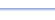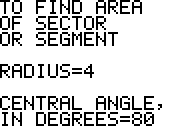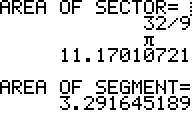Program: SECTOR

SECTOR

• Computes the area of a sector or segment of a circle, given the radius and central angle.
• Computes the length of an arc of a circle, given the radius and central angle.

Useful for:

• SAT
• ACT
• Geometry
• Precalculus
• Trigonometry
• Calculus

See full list of programs

Example

Q: Find (a)  the area of the shaded sector
in Diagram 1.
(b)  the length of arc AB in Diagram 1
(c)  the area of the shaded segment in Diagram 2

Solution:

Run SECTOR.  How?

Enter
CENTRAL ANGLE = 80

The program reports:

Press ENTER for more.
The program reports:

Press ENTER for more.
The program reports:

Interpretation: